# C++ Program to Demonstrate Use of Ternary Operator

In this tutorial you will learn about the C++ Program to Demonstrate Use of Ternary Operator and its application with practical example.

## C++ Program to Demonstrate Use of Ternary Operator

In this tutorial, we will learn to create a C++ program that will Demonstrate Use of Ternary Operator in C++ programming.

## Prerequisites

Before starting with this tutorial we assume that you are best aware of the following C++ programming topics:

• Operators in C++ Programming.
• Basic Input and Output function in C++ Programming.
• Basic C++ Programming.

## What is a Ternary Operator?

In the C++ programming language, the ternary operator is also known as the conditional operator and can be used to replace if…else conditional statements at some places.

## Program to Demonstrate Use of Ternary Operator:-

In this program, we will use the ternary operator instead of if…else conditional statement. For checking the input with the conditions and printing the input marks are in pass or fail criteria.

With the help of this program, we can take input and calcualtion.

## Output:-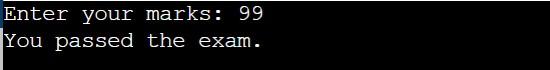In the above program, we have first initialized the required variable.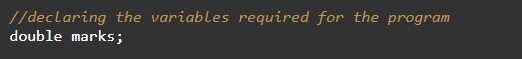• marks = it will hold the integer value.
• result= it will hold the string value.

Taking Input numbers from the user.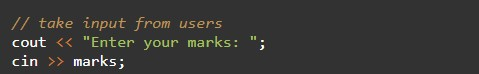Calculating the result.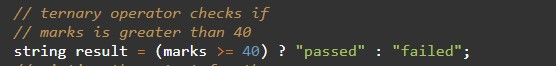Printing the output.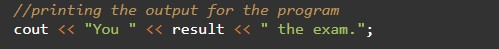In this tutorial we have learn about the C++ Program to Demonstrate Use of Ternary Operator and its application with practical example. I hope you will like this tutorial.